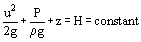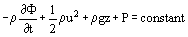# Bernoulli Equation

If the force-momentum equation is applied to an inviscid, incompressible fluid in steady flow, it may be shown that along any one streamtube:
(1)where u is the velocity, P is the pressure and z is the height above a predetermined datum. This equation expresses the conservation of mechanical work-energy and is often referred to as the incompressible steady flow energy equation or, more commonly, the Bernoulli equation, or Bernoulli’s theorem. All the quantities appearing within this equation have the physical dimensions of length and may be regarded as the energy per unit weight of fluid.
In hydraulic engineering, such quantities are referred to as “heads”, and the sum of all such terms as the total head. H. Bernoulli’s theorem expresses the conservation of total head along a given streamtube, and defines the balance between the kinetic energy represented by u2/2g, the potential energy, z, and the flow-work P/ρg, associated with the pressure forces.
The energy transfers described by Bernoulli’s theorem are all reversible (or conservative), and no account is taken of the nonreversible (or non-conservative) changes through which mechanical work-energy is transferred out of the system or converted to internal energy. In particular, the work done against the viscous shear stresses or external forces (other than gravity) are neglected. These omissions provide the greatest restriction to the applicability of Bernoulli’s theorem. All real fluids are to some extent viscous, and consequently, there will be some reduction in the total head due to the shear stress. This may be taken into account within Bernoulli’s theorem by incorporating a head loss (ΔE), which is either determined experimentally or assessed from previous engineering practice. Tabulated values of typical head losses in a wide variety of pipe flows and open channel flows are given by Idelchik (1986) in Zipparro and Hasen (1993).
In many practical cases, the energy loss (ΔE) will be small in comparison with other terms in Bernoulli’s equation. In particular, if the cross-sectional area of the flow reduces (i.e., the flow is convergent and therefore accelerates), the turbulence levels will tend to reduce. In this case, the energy losses are small and the changes in both the pressure and velocity are well predicted by Bernoulli’s theorem. If the flow diverges, however, the turbulence levels will increase and significant energy losses may result. In this case, Bernoulli’s theorem is inappropriate, and should not be applied.
The conservation of mechanical work-energy may also be applied to unsteady flows. Integration of the Euler equations produces the so-called unsteady Bernoulli equation. The most commonly used form of this equation arises when the flow is also irrotational (zero vorticity):
(2)where Φ represents the velocity potential. In this case, the equation is expressed in terms of the component pressures rather than energy heads. However, the limitations discussed previously still apply, and the equation should not be applied if there are significant nonconservative energy losses. A full derivation of the Bernoulli’s theorem, in both its steady and usteady form, is given by Duncan et al. (1970).